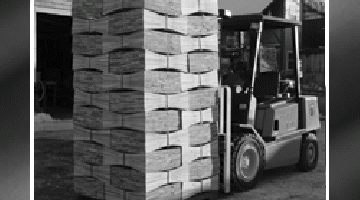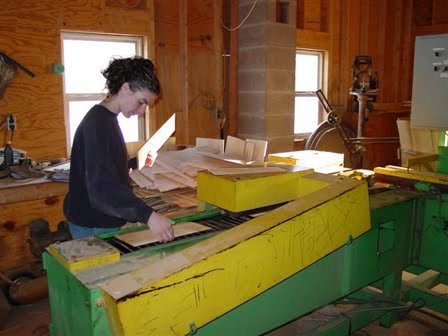Select PageHow many shingles do you need?

In order to determine how many cedar shingles are required to complete your project, we must determine the number of square feet of area you have to cover.  We must:

1. Measure the length and width of all surfaces to be covered. Figure appropriately for large windows and doors, keeping in mind that you may encounter a small amount of waste when siding these areas.
2. Multiply the length by the width of each side.
3. Add the totals for each side of the project.
4. Calculate the number of shingles needed.

Example:

A small barn with two walls measuring 20’ wide and 12’ high (Walls 1). There are also two end walls measuring 12’ wide and 12’ high (Walls 2). The gable ends are triangular, measuring 12’ wide and 4’ high (Gable Ends). No windows or doorways are expected.

Measurements:

Walls 1: 20’ x 12’ = 240 square feet x 2 = 480 square feet
Walls 2: 12’ x 12’ = 144 square feet x 2 = 288 square feet
Gable Ends: 12’ x 4’ divided by 2 = 24 square feet x 2 = 48 square feet

*Formula for calculating triangles: ½ base x height thus, one would purchase 8-1/2 square (34 bundles) of cedar shingles to cover this building.

Totaling the number of square feet, we have 816 square feet to be covered.

You would purchase 8-81/2 square (34 bundles) of cedar shingles to cover this building.

Click here for a printable form to guide you in estimating the amount of shingles you are going to need.